1. 北京航空航天大学 计算机学院, 北京 100085 ;
2. 湖北大学 计算机与信息工程学院, 武汉 430062 ;
3. 桂林电子科技大学 计算机科学学院, 桂林 541004

Program security verification based on abstract invariants
ZHANG Yan1,2 , QIAN Junyan3 , LI Zhoujun1, MA Dianfu1
1. School of Computer Science and Engineering, BeiHang University, Beijing 100085, China ;
2. School of Computer Science and Information Engineering, Hubei University, Wuhan 430062, China ;
3. Department of Computer science and Engineering, Guilin University of Electronic Technology, Guilin 541004, China
Abstract:Program security verification is a difficult issue with program invariants used for program security verification. Verification of whether a program has the correct temporal nature is converted into a problem solved at a fixed point. However, directly constructing an algorithm to solve the fixed point problem is very difficult. This paper presents a class of invariant construction methods based on an abstract interpretation framework to solve the structure method. Firstly, the Tableau method is used to construct the figure of the temporal formula based on the traditional invariant structure rules, with forward or backward state transitions to improve the invariant validation rules. Secondly, this paper presents an abstract state transition system based on an abstract interpretation framework and gives the abstract invariants validation rules. Finally, the fixed point theorem of abstract invariants is used with the forward or reverse transformations to solve the approximate fixed point in an automatic validation method based on abstract invariants of the program. Approximate widening and narrowing operations based on the abstract fixed point invariant verification algorithm accelerate the convergence.
Key words: abstraction interpretation     invariant verification     fixed point     program security verification

1 基础知识 1.1 转换系统

 \begin{align} & pre\left[ R \right]\left( X \right)\triangleq \left\{ s\left| \exists v.\left( s,v \right)\in R\wedge v\in X \right. \right\}, \\ & \widetilde{pre}\left[ R \right]\left( X \right)\triangleq C\circ pre\left[ t \right]\circ C= \\ & \left\{ s\left| \forall \right.v.\left( s,v \right)\in R\Rightarrow v\in X \right\}, \\ & post\left[ R \right]\left( X \right)\triangleq \left\{ v\left| \exists s.s\in X\wedge \left( s,v \right)\in R \right. \right\}, \\ & \widetilde{post}\left[ R \right]\left( X \right)=C\circ post\left[ t \right]\circ C= \\ & \left\{ v\left| \forall \right.s.\left( s,v \right)\in R\Rightarrow s\in X \right\}. \\ \end{align}

 \begin{align} & pre\left[ T \right]\left( X \right)\triangleq {{\cap }_{t\in T}}pre\left[ t \right]\left( X \right), \\ & post\left[ T \right]\left( Y \right)\triangleq {{\cap }_{t\in T}}post\left[ t \right]\left( t \right), \\ \end{align}

 $X\subseteq pre\left[ T \right]\Leftrightarrow post\left[ T \right]\left( X \right)\subseteq X.$
1.2 不变式验证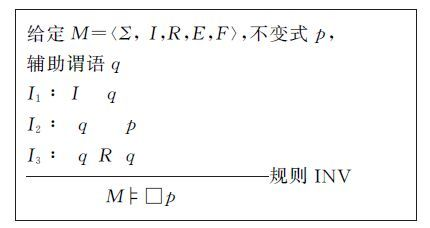图 1 规则INV

1) 标准的表示方法: φ∪ ∪{s′|(s,s′)∈t∧s ∈φ} ⊆ψ

2) 基于逆向转换的表示方法： $\bar{x}$.φ⊆ pre[t](ψ)；

3) 基于前向转换的表示方法： $\bar{x}'$. [post［t](φ)⊆ψ.

1.3 可达性与不动点

 $lf{{p}^{\sqsubseteq }}f=\left\{ \bigcap \left\{ x\in L\left| f\left( x \right)\subseteq x \right. \right\} \right.={{\bigcup }_{n\in \mathbb{N}}}{{f}^{n,\uparrow }}\left( \bot \right).$

1) n=0,f0,↑(x)=x

2) 后继序数n+1,fn+1,↑(x)=f(fn,↑(x))；

3) 有限序数n,fn,↑(x)=Ui<nfi,↑(x) .

 $gf{{p}^{\sqsubseteq }}f=\bigcup \left\{ x\in L\left| x\subseteq f\left( x \right) \right. \right\}={{\supset }_{n\in \mathbb{N}}}{{f}^{n,\downarrow }}\left( \text{T} \right)$

R*=∪i≥0Ri (其中R0$\triangleq${(s,s)|sΣ},且Rn+1$\triangleq$R$\circ$Rn= Rn$\circ$R)是二元关系R传递的、自反的闭包，则post[R*]I=lfpIλX.X ∪ post[R](X)=lfpλX.I ∪ post[R](X)。 通过不动点定理，lfpI λX.X ∪ post[R](X)求解如下： lfpI λX.X ∪ post[R](X)=∪i≥0Xi，其中X0=I,Xi+1= Xi ∪post[R](X)。

2 基于谓词图的不变式验证

2.1 谓词图

1) ㄱf1∈cl(φ) 当且仅当 f1∈cl(φ)；

2) 如果f1f2∈cl(φ)，那么f1,f2∈cl(φ)；

3) 如果X f1∈cl(φ)，那么f1∈cl(φ)；

4) 如果ㄱX f1∈cl(φ)，那么Xf1∈cl(φ)；

5) 如果f1 U f2∈cl(φ)，那么f1,f2,X [f1 U f2]∈cl(φ).

1) N⊆2cl(φ)(nN)当且仅当

a) φn

b) 对任何f∈cl(φ)，fn当且仅当ㄱfn

c) 对任何f1f2∈cl(φ)，f1f2n当且仅当f1nf2n

d) 对任何ㄱX f1∈cl(φ)，ㄱX f1n当且仅当Xf1n

e) 对任何f1Uf2∈cl(φ)，f1Uf2n，那么f1,X [f1Uf2]∈nf2n

2) N0N (nN0) 当且仅当φn

3) (n1,n2) ∈E当且仅当

a) 对任意X f1∈cl(φ)，X f1n1当且仅当f1n2

b) 对任意f1Uf2∈ cl(φ)，f1Uf2n1当且仅当f2n1f1Uf2n2

4) λ(n)=∧{ fn|f是一阶公式}。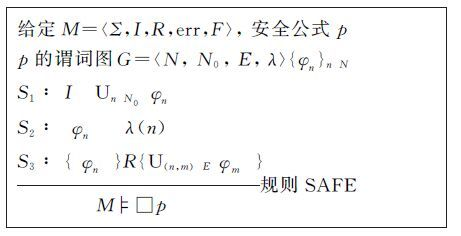图 2 规则SAFE

2.2 基于前向转换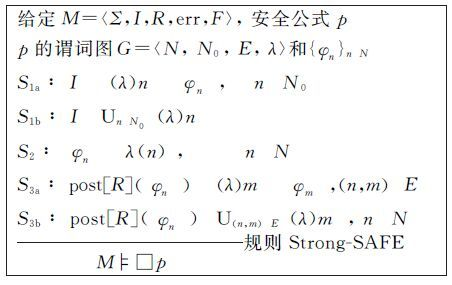图 3 规则Strong-SAFE

 \begin{align} & post\left[ R \right]\left( \left[\!\left[ {{\varphi }_{n}} \right]\!\right] \right)\subseteq {{\bigcup }_{\left( n,m \right)\in E}}\left[\!\left[ \lambda \left( m \right) \right]\!\right]\subseteq \\ & {{\bigcup }_{\left( n,m \right)\in E}}\left\{ post\left[ R \right]\left( \left[\!\left[ {{\varphi }_{n}} \right]\!\right] \right)\cap \left[\!\left[ \lambda \left( m \right) \right]\!\right] \right\}\subseteq \\ & {{\bigcup }_{\left( n,m \right)\in E}}\left[\!\left[ {{\varphi }_{m}} \right]\!\right] \\ \end{align}

 \begin{align} & F\left[ R \right]{{I}_{n}}\left( \left[\!\left[ {{\varphi }_{n}} \right]\!\right] \right)\triangleq \left[ \left( I\cap {{\left[\!\left[ n \right]\!\right]}_{n\in {{N}_{0}}}} \right) \right.\cup \\ & \left. {{\bigcup }_{\left( n,m \right)\in E}}\left\{ post\left[ R \right]\left( \left[\!\left[ {{\varphi }_{m}} \right]\!\right] \right) \right\} \right]\cap \left[\!\left[ \lambda \left( n \right) \right]\!\right]. \\ \end{align}

2.3 基于逆向转换

 \begin{align} & B\left[ R \right]{{P}_{n}}\left( {{\varphi }_{N}} \right)\triangleq \left[\!\left[ \lambda \left( n \right) \right]\!\right]\cap \\ & {{\bigcup }_{\left( n,m \right)\in E}}\left\{ pre\left[ R \right]\left( \left[\!\left[ {{\varphi }_{m}} \right]\!\right] \right) \right\},n\in N. \\ \end{align}

I⊆∪nN0[φB,n]，当且仅当p是状态有效的。从上向下验证p是状态有效的步骤如下：生成最大不动点，检查I⊆∪n∈N0[φB,n]是否满足。

3 状态系统抽象转换 3.1 抽象解释

3.2 构造抽象转换系统

1) ρM上的全映射；

2) 对任何状态s0,s1Σ且s0#Σ#,(s0,s0#)∈ρ，如果存在(s0,s1)∈R，那么存在状态s1#Σ#满足(s0#,s1#)∈R#且(s1,s1#)∈ρ；

3) 对在I中状态s，在I#中存在s#满足(s,s#)∈ρ.

1) ρM上的全映射；

2) 对任何状态s0,s1Σ且s0#Σ#,(s0,s0#)∈ρ，如果存在(s0,s1)∈R，那么存在状态s1#Σ#满足(s0#,s1#)∈R#且(s1,s1#)∈ρ；

3) IP

4) 对在I中状态s，在I#中存在s#满足(s,s#)∈ρ.

4 基于抽象的不变式验证规则图 4 抽象规则SAFE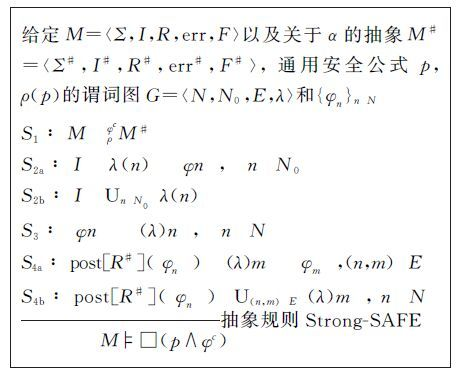图 5 抽象规则Strong-SAFE

5 基于抽象不动点的不变式验证

 \begin{align} & \forall X\subseteq \Sigma :\alpha \left( X\cup post\left[ R \right]\left( X \right) \right)\subseteq \\ & \alpha \left( X \right)\cup \left[ {{R}^{\#}} \right]\left( \alpha \left( X \right) \right). \\ \end{align}

 \begin{align} & \alpha \left( lf_{I}^{\subseteq }\lambda X.X\cup post\left[ R \right]\left( X \right) \right)\subseteq \\ & lf_{{{I}^{\#}}}^{\subseteq }\lambda X.X\cup post\left[ {{R}^{\#}} \right]\left( X \right). \\ \end{align}

 $post\left[ {{R}^{*}} \right]I\subseteq \gamma \left( lfp_{{{I}^{\#}}}^{\subseteq }\lambda X.X\bigcup post\left[ {{R}^{\#}} \right]\left( X \right) \right).$

 $post\left[ {{R}^{*}} \right]I\subseteq P$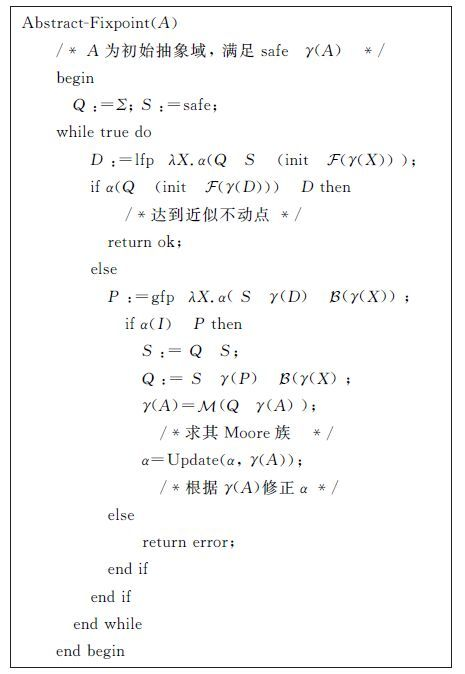图 6 基于抽象不动点检查的验证不变式算法

6 结 论

  Podelski A, Rybalchenko A. Transition invariants and transition predicate abstraction for program termination[C]//International Conference on Tools and Algorithms for the Construction and Analysis of Systems. Saarbrücken, Germany:Springer Verlag, 2011:3-10. http://cn.bing.com/academic/profile?id=1496222849&encoded=0&v=paper_preview&mkt=zh-cn  Bensalem S, Bozga M, Legay A, et al. Incremental component-based construction and Verification using invariants[C]//Proceedings of the IEEE/ACM Conference on Formal Methods in Computer-Aided Design. Lugano, Switzerland:IEEE Computer Society, 2010:257-266.  Chockler H, Ivrii A, Matsliah A, et al. Incremental formal verification of hardware[C]//Proceedings of the IEEE/ACM Conference on Formal Methods in Computer-Aided Design. Austin, Texas, USA:IEEE Computer Society, 2011:135-143.  Bensalem S, Legay A, Nguyen T. H, et al. Incremental invariant generation for compositional design[C]//Proceedings of The 4th Theoretical Aspects of Software Engineering Conference. Taipei, Taiwan, China:IEEE Computer Society, 2010:157-167.  I Dillig, T Dillig, B Li, et al. Inductive invariant generation via abductive inference[J]. Acm Sigplan Notices , 2013, 48 (48) : 443–456.  Lakhnech Y, Bensalem S, Berezin S. Incremental verification by abstraction[C]//Proceeding of the Tools and Algorithms for the Construction and Analysis of Systems. Genova, Italy:Springer Verlag, 2001:98-112. http://cn.bing.com/academic/profile?id=1515363397&encoded=0&v=paper_preview&mkt=zh-cn  Bj?rner N, Browne A, Manna Z. Automatic generation of invariants and intermediate assertions[J]. Theoretical Computer Science , 1997, 173 (1) : 49–87. DOI:10.1016/S0304-3975(96)00191-0  Bensalem S. Automatic generation of invariants[J]. Formal Methods in System Design , 1999, 15 (1) : 75–92. DOI:10.1023/A:1008744030390  Loiseaux C, Graf S, Sifakis J, et al. Property preserving abstractions for the verification of concurrent systems[J]. Formal methods in System Design , 1995, 13 (6) : 1–35.  Cousot P. Formal verification by abstract interpretation[C]//Proceedings of the 4th NASA Formal Methods Symposium. Norfolk, VA, USA:Springer Verlag, 2012:3-7.  Cousot P, Ganty P, Raskin F. Fixpoint-guided abstraction refinements[C]//Proceedings of the Fourteenth International Symposium on Static Analysis. Kongens Lyngby, Denmark:Springer Verlag, 2007:333-348. http://cn.bing.com/academic/profile?id=1514640333&encoded=0&v=paper_preview&mkt=zh-cn  Ranzato F, Rossi Doria, Tapparo F. A forward-backward abstraction refinement algorithm[C]//Proceedings of the 9th International Conference on Verification, Model Checking and Abstract Interpretation. San Francisco, CA, USA:Springer Verlag, 2008:248-262. http://link.springer.com/10.1007/978-3-540-78163-9_22  Gantya P, Maquetb N, Raskinb F. Fixed point guided abstraction refinement for alternating automata[J]. Theoretical Computer Science , 2010, 411 (38-39) : 3444–3459. DOI:10.1016/j.tcs.2010.05.037  Malkis A, Podelski A, Rybalchenko A. Thread-Modular Counterexample-Guided Abstraction Refinement[J]. Lecture Notes in Computer Science , 2011, 6337 : 356–372.  Meng W, He F, Wang B. Y, Liu Q. Thread-modular model checking with iterative refinement[C]//Proceedings of the NASA Formal Method. Berlin, Heidelberg:Springer-Verlag, 2012:237-251. http://cn.bing.com/academic/profile?id=102496483&encoded=0&v=paper_preview&mkt=zh-cn  Bensalem S, Graf S, Lakhnech Y. Abstraction as the key for invariant verification[J]. Lecture Notes in Computer Science , 2003, 2772 : 67–99. DOI:10.1007/b12001  Gulwani S, Srivastava S, Venkatesan R. Constraint-based invariant inference over predicate abstraction[J]. Lecture Notes in Computer Science , 2009, 5403 : 120–135. DOI:10.1007/978-3-540-93900-9  Patrick C, Radhia C. Parsing as abstract interpretation of grammar semantics[J]. Theoretical Computer Science , 2003, 290 : 531–544. DOI:10.1016/S0304-3975(02)00034-8